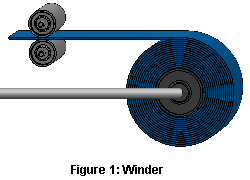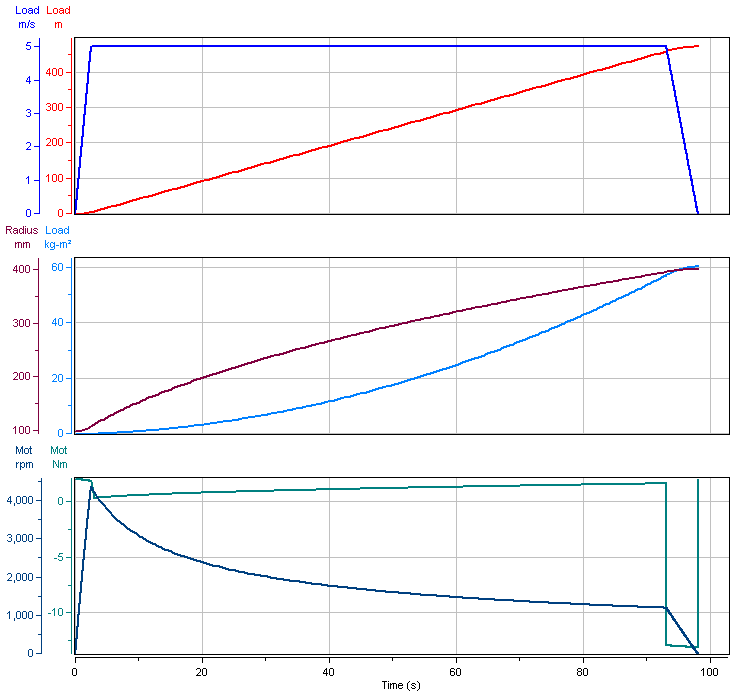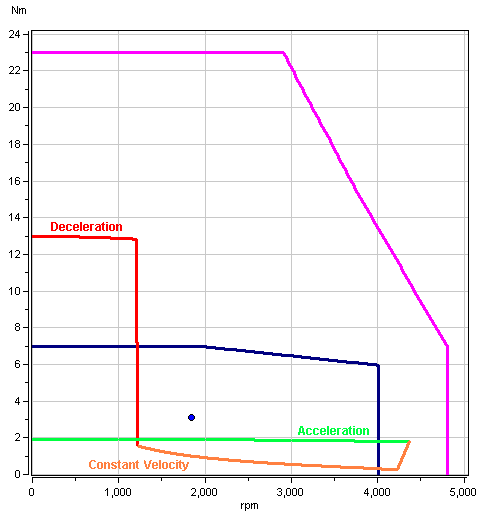## Sizing Winder Applications: The Need for an Accurate ModelMost sizing tools assume the winder is full of material at the maximum radius. When winding, this is the worst case scenario for decelerating the maximum inertia. In winding applications, most often the goal is to maintain a certain web tension at a constant linear speed. If the empty radius is say 25% of the full radius, then the motor and gearbox speeds can be up to 4x faster during acceleration than deceleration. This can lead to a motor and gearbox selection error by not accounting for the much faster motor and gearbox speeds during the acceleration phase.

Consider a winding application as shown in Figure 1 where the roller radius is 100mm, and when full of material, the effective radius grows to 400mm. The material speed is 5m/s, and the web tension is 50N. The gearbox ratio is 10:1. Figure 2 illustrates the accurate dynamic model of the entire winding sequence:

The top two profiles are the material linear velocity and length of wound material
The middle two profiles are the effective radius and the load inertia
The bottom two profiles are the motor speed and motor torque
At the start of acceleration, the roller is without material. The effective radius is only the roller radius, and the load inertia is small. Therefore, the motor speed ramps up to 4,360rpm to achieve the 5m/s material linear speed. As the winder fills with material, the radius inceases and the motor speed gradually decreases to maintain the material linear speed of 5m/s. As the deceleration segment begins, the motor speed is 1,200rpm.Figure 2: Winder Sequence

Figure 3 illustrates how the winder sequence translates to motor torque and speed. During acceleration (green segment), motor speed is the key design consideration. However, during deceleration (red segment), the motor torque is the key design consideration.Figure 3: Motor Torque vs Speed

Finally, for unwinding applications, the same issues occur, just in reverse. During acceleration, motor torque is a key design consideration. During deceleration, motor speed is an important design consideration.

The main point here is that an accurate winder model makes it easy for engineers to make smart design decisions for motor and gearbox selection based on the entire winder sequence. The engineer can study any portion of the profile, and then choose to adjust the profile, change the gearbox or choose a different motor. The model is performing all the detailed calculations, and the designer can easily evaluate numerous options depending on the design objectives.